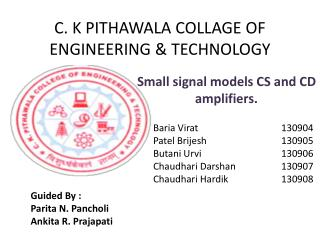# C. K PITHAWALA COLLAGE OF ENGINEERING & TECHNOLOGY - PowerPoint PPT PresentationDownload PresentationC. K PITHAWALA COLLAGE OF ENGINEERING & TECHNOLOGY

C. K PITHAWALA COLLAGE OF ENGINEERING & TECHNOLOGYDownload Presentation## C. K PITHAWALA COLLAGE OF ENGINEERING & TECHNOLOGY

- - - - - - - - - - - - - - - - - - - - - - - - - - - E N D - - - - - - - - - - - - - - - - - - - - - - - - - - -
##### Presentation Transcript

1. C. K PITHAWALA COLLAGE OF ENGINEERING & TECHNOLOGY BariaVirat 130904 Patel Brijesh 130905 ButaniUrvi 130906 ChaudhariDarshan 130907 ChaudhariHardik 130908 Small signal models CS and CD amplifiers. Guided By : Parita N. Pancholi Ankita R. Prajapati

2. Basic JFET Amplifiers • The JFET also can be used for the amplification of a small time varying signal. JFAT amplifier configurations : • Common source (CS) configuration • Common drain (CD) configuration

3. CS JFET Amplifiers • Circuit description : • In the circuit diagram of fig (a) an n-channel JEFT is being used for amplification. • The fixed biasing arrangement is used. The voltage source VGG will reverse bias the gate to source junction of the JEFT. • C1 and C2 are the coupling capacitors C1 will couple the AC input voltage to the gate of the JEFT and isolate the DC voltage VGG from the AC input while C2 will couple only the AC voltage to the load and block the DC voltage. • RD is the external resistance connected in the drain circuit to limit the drain current below a safe value.

4. Fig (a) Amplifier using n-channel JFET

5. Operation as amplifier • The AC signal which is to be amplified is applied at the input of the JFET amplifier. This AC signal will get superimposed on the negative DC bias voltage as shown in figure. (b). • In the positive half cycle of the input AC signal (0 to π) the gate to source voltage becomes less negative. This will increase the channel width and increase the level of drain current ID. Thus ID will very sinusoidally above its W point value as shown in fig (b). • The drain to source voltage Vps is given by • VDS = VDD- ID RD • Therefore as ID increases. The voltage drop ID RD will also increase and the voltage VDS will decrease as shown in fig (b). • VDS Reduce sinusoidally below its Q point value.

6. Fig (b) Waveform for a JEFT amplifier

7. Fig (c) : Amplification represented by the graphical representation At point A : ID = VDD / RD At point B : VDS = VDD Slop of the load line will be – 1/ R

8. Phase reversal • Referring to Fig (b) we can conclude that the AC output voltage is 180◦ out of phase with the AC input voltage. Thus there is a complete phase reversal. This is identical to the phase reversal obtained for the CE transistor amplifer. Features of CS amplifier • High input resistance • Low output resistance • High voltage gain • 180◦ phase shift between the input and output Application of CS amplifier • As a per-amplifier in audio circuit • As a voltage amplifier • In the PA system • In the radio and TV amplifier circuits.

9. Common Drain (CD) configuration • The JFET equivalent of the BJT emitter follower configuration is the source follower or common drain configuration as shown in fig (a). • The output is taken off the source terminal and in the AC equivalent circuit the drain appears to be grounded. Therefore the name common drain configuration. • Self biasing circuit is employed for the CD amplifier. • The input signal is applied at gate with respect to ground and the output is obtained at source. • In the ac equivalent circuit the drain (which is connected to VCC) gets connected to ground. So it becomes a common point between the input and output and this configuration is called as common drain configuration.

10. The input and output voltage waveforms for a CD amplifier are shown in Fig (b). • From the waveforms we can observe that the output voltage is equal to the input voltage and the difference between them is zero. • As the source voltage follows the input voltage . This circuit is called as source follower.

11. Fig (a) JFET common drain configuration

12. Fig (2) : Input output waveform

13. Features of CD amplifier • Low voltage gain • Very high input resistance • Very low output resistance • No phase shift between input and output

14. The End&Thank you